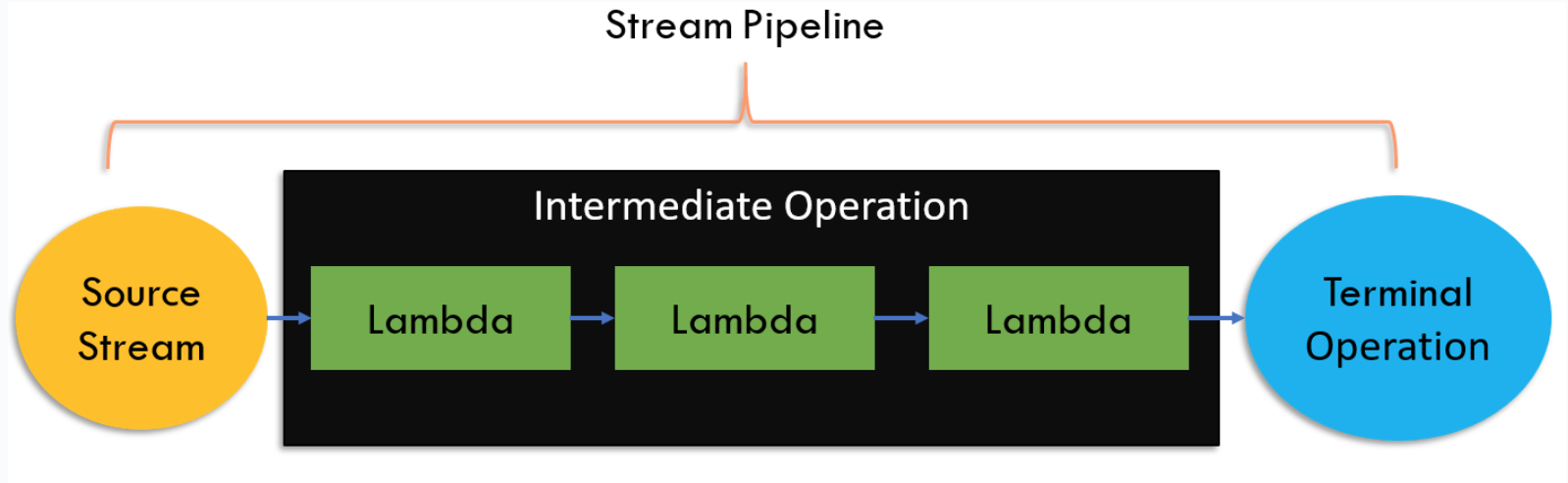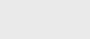2年前 (2021-10-02) |   抢沙发  283

[收起] 文章目录

Streams允许我们以声明的方式对数据集合进行计算。在一种声明式编程方式中，您不指定如何做，而是指定要做什么。您可以创建流管道来执行计算。流管道包括：

• 消息来源
• 零个或多个中间操作
• 终端操作

Java streams流是懒惰的。因此，仅当终端操作启动时才执行中间操作。

## Java streams pipeline 流管道

``````List<Book> books =
Catalog.books();

List<String> javaAuthors =
books.stream()
.filter(book -> book.getCategory().equals(JAVA))
.map(Book::getAuthor)
.collect(Collectors.toList());
``````

### 终端操作### 创建stream流

#### 来自单个元素的流

`Stream of(T… values)`–返回元素为指定值的顺序流。

``````Stream<String> numbers =
Stream.of("One", "Two", "Three");``````

`ofNullable(T t)`–返回包含单个元素的顺序流，如果非空，则返回空流。

``````Stream<String> numbers =
Stream.ofNullable("One");

Stream<String> empty =
Stream.empty(); ``````

``````static Stream<Integer> toStream(Collection<Integer> numbers) {
return Stream.ofNullable(numbers)
.flatMap(Collection::stream);
}``````

Java9中添加了`nullable（T）`方法。

``````return Optional
.ofNullable(numbers)
.stream()
.flatMap(Collection::stream);
``````

### 来自数组的流

``````String[] names =
new String[] {"Jack","Jill"};

Stream<String> stream1 =
Stream.of(names);

Stream<String> stream2 =
Arrays.stream(names);``````

### 来自集合的流

``````List<String> namesList =
List.of("Jack", "Jill");

Stream<String> stream =
namesList.stream();
``````

### 来自Builder的流

``````Stream<String> stream =
Stream.<String>builder()
.build();``````

### 流使用Generate

``````Stream<Integer> randomNumbers =
Stream
.generate(new Random()::nextInt)
.limit(10);``````

### 使用迭代的流

``````Stream<Integer> even =
Stream
.iterate(0, n -> n + 2)
.limit(10);
``````

### 原始流

``````@Setter
@Getter
public class Book {
private String name;
private Category category;
private double price;
private String author;
private String publisher;
}``````

``````static double priceOfAllBooks(List<Book> books) {
Double allBooksPrice =
books.stream()
.map(Book::getPrice)
.reduce(0d, Double::sum);

return allBooksPrice;
}
``````

``````static double priceOfAllBooks(List<Book> books) {
double allBooksPrice =
books.stream()
.mapToDouble(Book::getPrice)
.sum();

return allBooksPrice;
}       ``````

Java有三个基本流–`IntStream``DoubleStream``LongStream`来执行流操作`int``double``long`基本类型。

### 创建原始流

#### Of

``````IntStream intOne =
IntStream.of(1);

IntStream intOneTwo =
IntStream.of(1,2);

DoubleStream doubleOne =
DoubleStream.of(1);

LongStream longOneTwo =
LongStream.of(1,2); ``````

#### 范围

``````IntStream oneToNine =
IntStream.range(1, 10);

// Range inclusive
IntStream oneToTen =
IntStream.rangeClosed(1, 10);``````

#### Generate

``````IntStream tenOnes =
IntStream.generate(() -> 1).limit(10);

DoubleStream tenRandomDouble =
DoubleStream
.generate(() -> new Random()
.nextDouble()).limit(10);
``````

#### 斐波那契数的生成

``````public class Fibonacci {
private int prev = 0;
private int curr = 1;

private int next() {
int temp = prev + curr;
prev = curr;
curr = temp;
return curr;
}

public IntStream stream() {
return IntStream
.generate(this::next);
}
}

//Caller
Fibonacci fibonacci =
new Fibonacci();

IntStream fibStream =
fibonacci.stream().limit(10);``````

#### 迭代

``````IntStream evenNumbers =
IntStream
.iterate(0, n -> n + 2)
.limit(10);``````

## 创建流的其他方法

### 连接两个字符串

``````Stream<Integer> prime =
Stream
.concat(Stream.of(2, 3),
Stream.of(5, 7, 11));
``````

String

Java8添加了实用程序方法来从String对象创建流。

Lines

``````static Stream<String> lines() {
return "Line separated by newline.\nAnother line."
.lines();
}     ``````

1. 由换行符分隔的行

2. 另一行

### Chars

``````static IntStream chars() {
return "XYZ".chars();
} ``````

### Random

`Random`类有两个有用的方法来生成原始专门化类型的随机数流。

``````// Infinite Stream
IntStream randomInts =
new Random().ints();

// Fixed Size Stream
IntStream tenRandomInts =
new Random().ints(10);

// Infinite Stream
DoubleStream randomDoubles =
new Random().doubles();

// Fixed Size Stream
DoubleStream tenRandomDoubles =
new Random().doubles(10);

// Infinite Stream
LongStream randomLongs =
new Random().longs();

// Fixed Size Stream
LongStream tenRandomLongs =
new Random().longs(10); ``````

## 总结

Streams允许我们以声明的方式对数据集合进行计算。您可以创建流管道来执行计算。流管道包括：

• 消息来源
• 零个或多个中间操作
• 终端操作

Java8还添加了专门的原语流。例如，`IntStream``DoubleStream``LongStream`都是原始流。### 注册